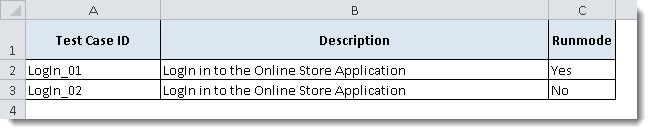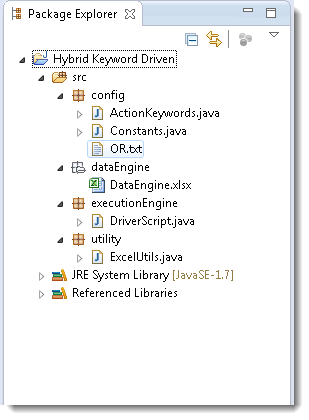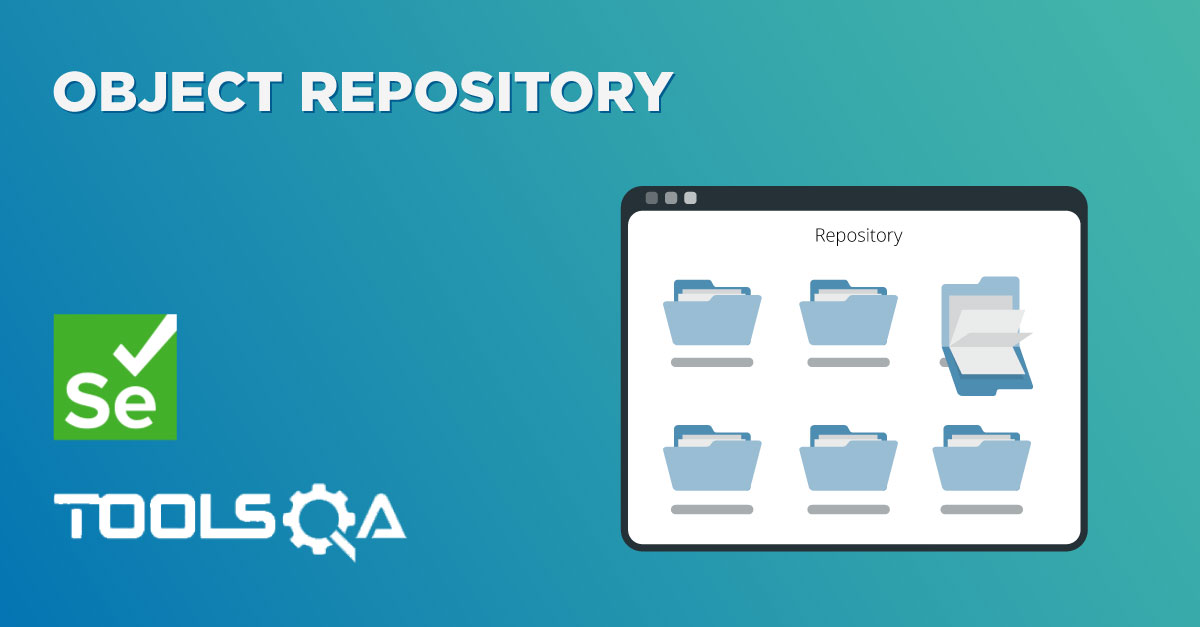When we talk about Framework, we think of getting work done much efficiently and more effectively. In the current stage of our framework, we are just running a single test case. In a real-world scenario, it can be hundreds of test cases or can be a number of test suites. Plus there can be the need for executing only a few test cases from the test suite. A decent framework should cater all of these requirements

In this chapter, we will learn how to execute test case one by one as a Test Suite. So that multiple test cases can be executed together. As we have taken excel for the data input source, it is better to drive rest of the things from the excel only.

## Step 1: Create a sheet for Test Cases

1. Create a new sheet in the same 'DataEngine' excel file and name it as 'Test Cases'.
2. Create three columns in its naming 'Test Case ID', 'Description', 'Run Mode'.
3. Test Case ID can be like LogIn_001, LogIn_002 and so on or anything of your choice.
4. Description is the small one-liner description of the test case.
5. Run Mode states that the test needs to be executed or not, so it can be 'Yes' or 'No'

### Data Engine file:## Step 2: Update Constants file

1. Create constant variables for new columns (Test Case ID & Run Mode ) of the Test Cases sheet
2. Create a contact variable for Test case sheet as well.

### Constants Variable Class:

``````package config;

public class Constants {

//List of System Variables
public static final String URL = "https://www.store.demoqa.com";
public static final String Path_TestData = "D://Tools QA Projects//trunk//Hybrid KeyWord Driven//src//dataEngine//DataEngine.xlsx";
public static final String Path_OR = "D://Tools QA Projects//trunk//Hybrid KeyWord Driven//src//config//OR.txt";
public static final String File_TestData = "DataEngine.xlsx";

//List of Data Sheet Column Numbers
public static final int Col_TestCaseID = 0;
public static final int Col_TestScenarioID =1 ;
public static final int Col_PageObject =3 ;
public static final int Col_ActionKeyword =4 ;
//New entry in Constant variable
public static final int Col_RunMode =2 ;

//List of Data Engine Excel sheets
public static final String Sheet_TestSteps = "Test Steps";
//New entry in Constant variable
public static final String Sheet_TestCases = "Test Cases";

//List of Test Data
public static final String UserName = "testuser_3";
public static final String Password = "[email protected]";

}
``````

Note: If you notice that no new constant variable is created for the new column 'Test Case ID' for the sheet 'Test Cases'. It is just because that the 'Test Case ID' column is also exists in 'Test Step' sheet and also at the same column number, so there is no need to create new, as one can used in both the places.

## Step 3: Modify ExcelUtils class

1. Modify method 'setExcelFile' of class ExcelUtils, as of now it is taking two arguments Data Engine Path & Excel Sheet Name. Now we have two different sheets, one is Test Cases and other is Test Steps, so we will be providing sheet name as an extra argument with all the Excel functions and hence it is better to remove Excel Sheet Name argument from 'ExcelUtils' function.
2. Modify function 'getCellData', as discussed above now all the 'Excelutils' methods will have an extra argument Sheet Name.
3. Create a new method 'getRowContains', this method will have three arguments, first Test Case Name, second Column Number and third Sheet Name. This will return the row number of the current test case.
4. Create a new method 'getTestStepsCount', this will again have three arguments. First Test Case ID, second Test Case first step and third Sheet Name. This function will return the count of test steps of a test case.

### Excel Utility Class:

``````package utility;

import java.io.FileInputStream;

import org.apache.poi.xssf.usermodel.XSSFCell;
import org.apache.poi.xssf.usermodel.XSSFSheet;
import org.apache.poi.xssf.usermodel.XSSFWorkbook;

import config.Constants;
public class ExcelUtils {
private static XSSFSheet ExcelWSheet;
private static XSSFWorkbook ExcelWBook;
private static XSSFCell Cell;
//private static XSSFRow Row;

//This method is to set the File path and to open the Excel file
//Pass Excel Path and SheetName as Arguments to this method
public static void setExcelFile(String Path) throws Exception {
FileInputStream ExcelFile = new FileInputStream(Path);
ExcelWBook = new XSSFWorkbook(ExcelFile);
}

//This method is to read the test data from the Excel cell
//In this we are passing Arguments as Row Num, Col Num & Sheet Name
public static String getCellData(int RowNum, int ColNum, String SheetName ) throws Exception{
ExcelWSheet = ExcelWBook.getSheet(SheetName);
try{
Cell = ExcelWSheet.getRow(RowNum).getCell(ColNum);
String CellData = Cell.getStringCellValue();
return CellData;
}catch (Exception e){
return"";
}
}

//This method is to get the row count used of the excel sheet
public static int getRowCount(String SheetName){
ExcelWSheet = ExcelWBook.getSheet(SheetName);
int number=ExcelWSheet.getLastRowNum()+1;
return number;
}

//This method is to get the Row number of the test case
//This methods takes three arguments(Test Case name , Column Number & Sheet name)
public static int getRowContains(String sTestCaseName, int colNum,String SheetName) throws Exception{
int i;
ExcelWSheet = ExcelWBook.getSheet(SheetName);
int rowCount = ExcelUtils.getRowCount(SheetName);
for (i=0 ; i<rowCount; i++){
if  (ExcelUtils.getCellData(i,colNum,SheetName).equalsIgnoreCase(sTestCaseName)){
break;
}
}
return i;
}

//This method is to get the count of the test steps of test case
//This method takes three arguments (Sheet name, Test Case Id & Test case row number)
public static int getTestStepsCount(String SheetName, String sTestCaseID, int iTestCaseStart) throws Exception{
for(int i=iTestCaseStart;i<=ExcelUtils.getRowCount(SheetName);i++){
if(!sTestCaseID.equals(ExcelUtils.getCellData(i, Constants.Col_TestCaseID, SheetName))){
int number = i;
return number;
}
}
ExcelWSheet = ExcelWBook.getSheet(SheetName);
int number=ExcelWSheet.getLastRowNum()+1;
return number;
}

}
``````

## Step 4: Write code to drive execution from the Excel sheet

1. Set up an outer 'for loop' on the Test Case Id column of the Test Cases sheet, which runs from first test case to the last test case of the sheet.
2. Value from Run Mode column decides the execution of the test case.
3. Set up an another inner 'for loop' on the Test Case ID column of the Test Step sheet, which runs from the first test step of the current test case to the last test step.

### Driver Script Class:

``````package executionEngine;

import java.io.FileInputStream;
import java.lang.reflect.Method;
import java.util.Properties;

import config.ActionKeywords;
import config.Constants;
import utility.ExcelUtils;

public class DriverScript {

public static Properties OR;
public static ActionKeywords actionKeywords;
public static String sActionKeyword;
public static String sPageObject;
public static Method method[];

public static int iTestStep;
public static int iTestLastStep;
public static String sTestCaseID;
public static String sRunMode;

public DriverScript() throws NoSuchMethodException, SecurityException{
actionKeywords = new ActionKeywords();
method = actionKeywords.getClass().getMethods();
}

public static void main(String[] args) throws Exception {
ExcelUtils.setExcelFile(Constants.Path_TestData);

String Path_OR = Constants.Path_OR;
FileInputStream fs = new FileInputStream(Path_OR);
OR= new Properties(System.getProperties());

DriverScript startEngine = new DriverScript();
startEngine.execute_TestCase();
}

private void execute_TestCase() throws Exception {
//This will return the total number of test cases mentioned in the Test cases sheet
int iTotalTestCases = ExcelUtils.getRowCount(Constants.Sheet_TestCases);
//This loop will execute number of times equal to Total number of test cases
for(int iTestcase=1;iTestcase<=iTotalTestCases;iTestcase++){
//This is to get the Test case name from the Test Cases sheet
sTestCaseID = ExcelUtils.getCellData(iTestcase, Constants.Col_TestCaseID, Constants.Sheet_TestCases);
//This is to get the value of the Run Mode column for the current test case
sRunMode = ExcelUtils.getCellData(iTestcase, Constants.Col_RunMode,Constants.Sheet_TestCases);
//This is the condition statement on RunMode value
if (sRunMode.equals("Yes")){
//Only if the value of Run Mode is 'Yes', this part of code will execute
iTestStep = ExcelUtils.getRowContains(sTestCaseID, Constants.Col_TestCaseID, Constants.Sheet_TestSteps);
iTestLastStep = ExcelUtils.getTestStepsCount(Constants.Sheet_TestSteps, sTestCaseID, iTestStep);
//This loop will execute number of times equal to Total number of test steps
for (;iTestStep<=iTestLastStep;iTestStep++){
sActionKeyword = ExcelUtils.getCellData(iTestStep, Constants.Col_ActionKeyword,Constants.Sheet_TestSteps);
sPageObject = ExcelUtils.getCellData(iTestStep, Constants.Col_PageObject, Constants.Sheet_TestSteps);
execute_Actions();
}
}
}
}

private static void execute_Actions() throws Exception {

for(int i=0;i<method.length;i++){
if(method[i].getName().equals(sActionKeyword)){
method[i].invoke(actionKeywords,sPageObject);
break;
}
}
}
}
``````

Note: For any explanation on above non commented code, please refer previous chapters.

### Project folder in Eclipse will look like this now:ReviewersVirender Singh